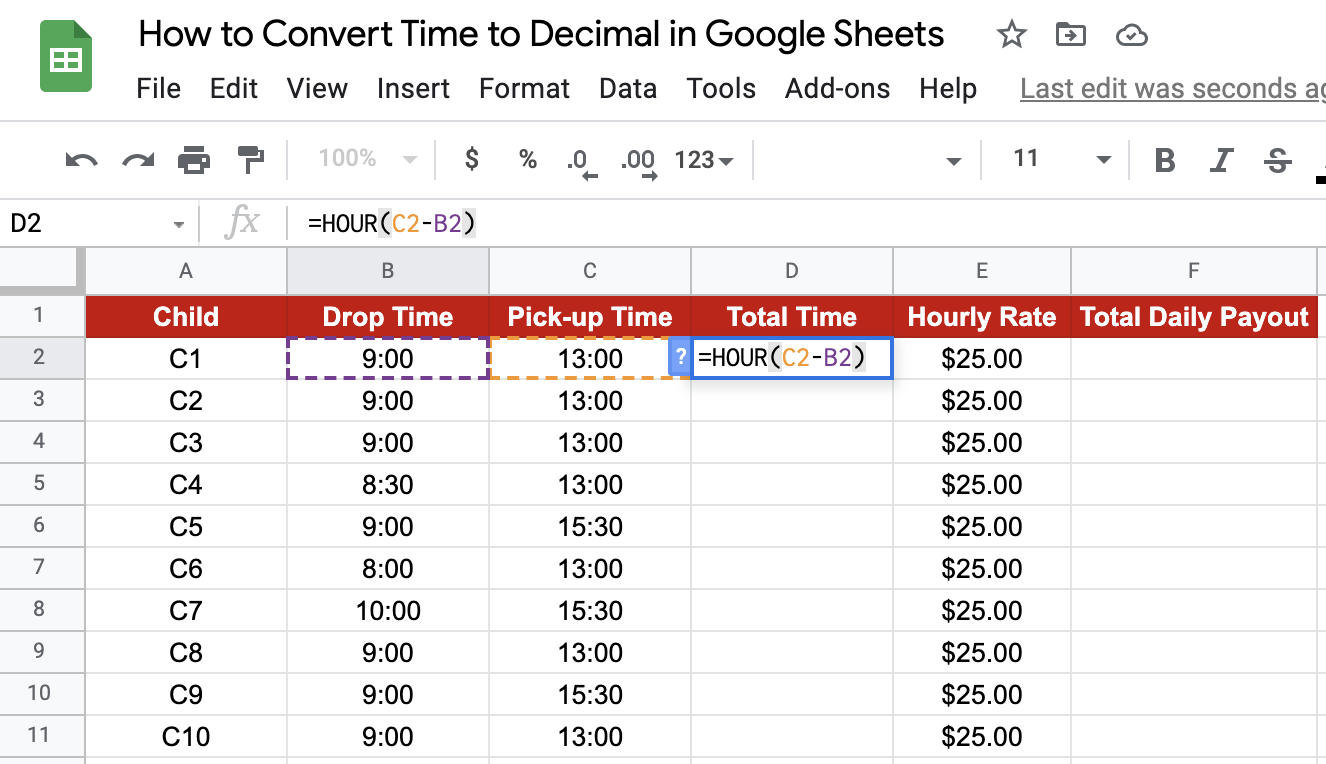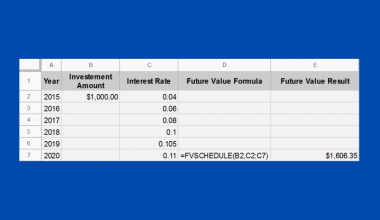# How to Convert Time to Decimal in Google Sheets

At times, you may run into a situation where you need to convert time to a decimal number in Google Sheets. Let’s say you want the difference between two-time instances. It might be more intuitive to say that the difference is 3.25 hours rather than to say 3 hours and 15 minutes. This is especially important if you need to use this difference in subsequent calculations, such as total wages based on hours worked and hourly wage.

So how do you do it? There are a couple of methods by which we can achieve this desired result in Google Sheets: using `TIME()`functions (`HOUR()`, `MINUTE()`, and `SECOND()`) or simple multiplication with `TIMEVALUE()`. We’ll take a look at both of them in this guide.

Let’s take an example.

## A Real Example of Converting Time to Decimal

A construction supervisor has the following rap sheet with working hours for contractors under him during the first half of a given day:The column D calculates the hours a given contractor has put in for the day. This will be used further in calculating the total wage payable to the contractor for the day. A simple subtraction will not give a result that can be used for further calculation. How to proceed, then?

There are two ways we can calculate the difference that results in a number output. Either using the `HOUR()` function or the `TIMEVALUE()` function. The `HOUR()` function returns the difference as a measure of the hour of the day. For example, 11:00-9:00 is represented as 2 hours. Similarly, the same difference as an output of the `TIMEVALUE()` function will represent a fraction of the day. For e.g., 11:00-9:00 will give 0.083 (2 hours is 0.083 of a day). The output obtained using these two formulas is as below:As you see above, we can now directly calculate the total payout (based on an hourly wage or a daily wage). You play around with the time values and verify if the results get correctly populated. Copy the spreadsheet using the link below:

Amazing! Let’s take a closer look at the components of the function and then move on to a detailed walkthrough of using `TIME()` and `TIMEVALUE()` functions to convert time into decimal values.

## The Anatomy of the Functions

The first method is to use the `TIME()` functions (`HOUR()`, `MINUTE()`, and `SECOND()`). We write the `HOUR()` function (for example) as given below:

`=HOUR(time)`

Let us look at what each term means:

• HOUR denotes our `HOUR()` function.
• time is the data that provides the time representation.

The `MINUTE()` and `SECOND()` functions follow the exact same anatomy, with corresponding output in terms of minutes and seconds, respectively.

The second method is to use the `TIMEVALUE()` function. The syntax (the way we write) of the `TIMEVALUE()` function is as follows:

Let us look at what each term means:

• TIMEVALUE denotes our `TIMEVALUE()` function.
• time_string is the data that provides the time representation.

## How to Convert Time to Decimal in Google Sheets: Time Functions

1. Below are the drop-off and pick-up times for kids at a créche facility. The parents are billed hourly based on the time the kid spends at the facility. The objective is to calculate the total time spent as a difference between pick-up and drop time and use the same to calculate the total daily payout based on the hourly rate.1. Now, simply click on any cell to make it the active cell. For this guide, I will be selecting D2, where I want to show my results.
2. Next, type the equal sign ‘=‘ to begin the function, followed by the name of the function, which is our `HOUR()`.
3. An auto-suggest box will appear along with the function name. Proceed to entering the opening bracket ‘(‘  as given below:1. Now, the fun begins! Let’s give the required inputs to the function to get the time difference in numeric form. Note that we are giving C2B2 as the input to the function since we are interested in the difference:1. To accurately capture the total time, we use the `MINUTE()` and `SECOND()` function along with `HOUR()` in the exact same way:

HOUR(C2-B2) + (MINUTE(C2-B2)/60) + (SECOND(C2-B2)/3600).
2. After entering the required inputs to the function, close the brackets and press the Enter key. You will notice that the difference in time is output as the number of hours. The total payout is the product of columns D and E.## How to Convert Time to Decimal in Google Sheets: Timevalue function

1. We will use the same example as above. The objective remains the same.
2. To enter the `TIMEVALUE()` function, click on a new cell, say G2.
3. Same as above, type the equal sign ‘=‘ to begin the function and then followed by the name of the function, which is our `TIMEVALUE()`.4. Let’s now give the required inputs to the function to get the time difference in numeric form. Note that we are giving C2B2 as the input to the function since we are interested in the difference:1. Close the brackets after you have input the required parameters to the function and press the Enter key. You will notice that the difference in time is output as a decimal which represents a portion of a day. For example, 4 hours is output as 0.167. The total payout is calculated as a product of columns G and E,  further multiplied by 24 since we have an hourly rate.There you go. You are now ready to start with converting time to decimal in Google Sheets. I recommend experimenting with different time values and some of the other Google Sheets formulas available together, to see how the results differ.Our goal this year is to create lots of rich, bite-sized tutorials for Google Sheets users like you. If you liked this one, you'll love what we are working on! Readers receive ✨ early access ✨ to new content.

##### You May Also Like## How to Use RANK.AVG Function in Google Sheets

The RANK.AVG function in Google Sheets is used to determine the rank of a specific value in a…## How to Use DSTDEVP Function in Google Sheets

The DSTDEVP function in Google Sheets is useful if you need to calculate the standard deviation of a…## How to Use FVSCHEDULE Function in Google Sheets

The FVSCHEDULE function in Google Sheets is useful when you want to know the future value of an…## How to Remove Extra Characters from Strings in Google Sheets

Removing repeated characters from the end of strings in your Google Sheets document makes it much easier to…